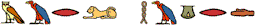Zen Pharaohs of Ultimate Love mathematics, music, science, wood....what else could you ask?Up

## Available Publications

### Postscript Files:

The adaptive identification of the impulse response of an innovation filter is considered. The impulse response is a finite sum of known basis functions with unknown coefficients. These unknown coefficients are estimated using a pseudolinear regression. This estimate is implemented using a square root algorithm based on a displacement rank structure. When the initial conditions have low displacement rank, the filter update is O(n). If the filter architecture is chosen to be triangular input balanced, the estimation problem is well-conditioned and a simple, low rank initialization is available.

The condition number of the nxn matrix P is examined, where P solves Stein's equation, P-APA*=BB*, where B is a nxd matrix. A simulation study shows that P is generically ill-conditioned in the case of n>>1 and d=1. Lower bounds on the condition number of P are given when A is normal, a single Jordan block or in Frobenius form. The bounds show a similar ill-conditioning for n/d>>1. These bounds are related to the condition number of the transformation that takes A to triangular input balanced form.

The matrix equation MA=N is considered. The lgrade of a matrix A is the the largest rank of any subdiagonal block of a symmetric partition of a square matrix. When A has lgrade d, representation results are given for MA=N with M and N having lower bandwidth d. M can be chosen to be lower triangular or unitary. A second result is that if MA=N with M and N have lower bandwidths dM and dN then A=U+V with the lwidth(U)=dN-dM and U is an upper triangular matrix. If A has lower bandwidth d and upper grade d, then A has a representation as the product of n-d-1 matrices
each of which is the identity except for a diagonal subblock of size d +1

### Cocktail Party Napkins Section:

Recent studies using electron transport in polymers suggest a Markov model for quantum wires. The pictures demonstrate that the model is in qualitative agreement with the observed phenomena; where the tunneling mode dominates transport in the short (small number of state) cases, but drift-diffusion dominates the longer bridge (larger number of states) cases. As expected, the intermediate lengths combine both modes of electron transport.

### Other Authors' Useful Work

Memory Mapped Files: An important programming technique that seems to still have gone largely unnoticed!

A paper of Trefethen and Howle of interest to musicians, among other instruments, guitars are treated.